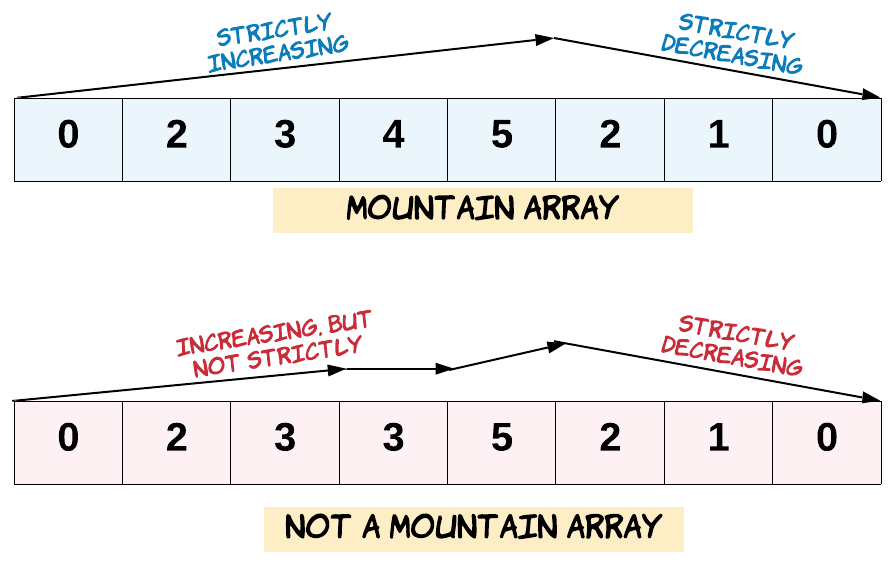### 问题描述

• A.length >= 3
• 在 0 < i < A.length - 1 条件下，存在 i 使得：
• A < A < ... A[i-1] < A[i]
• A[i] > A[i+1] > ... > A[A.length - 1]```输入：[2,1]

```

```输入：[3,5,5]

```

```输入：[0,3,2,1]

```

• 0 <= A.length <= 10000
• 0 <= A[i] <= 10000

### 解题思路

• state 表示处于上升状态（0），还是下降状态（1），初值为 0
• left 表示山顶左面的长度，初值为 0
• right 表示山顶右面的长度，初值为 0

• 如果它大于前面的元素，并且 state 等于 0，那么说明正在上升，将 left 增加 1；如果 state 等于 1，那么说明在下降阶段，遇到了上升的情形，返回 false
• 如果它等于前面的元素，那么返回 false
• 否则如果state 等于 0，那么说明前面的元素是山顶，将 state 置为 1，从此时起进入到下降阶段；如果 state 等于 1，那么说明正在下降。然后将 right 增加 1

### Python 实现

```class Solution(object):
def validMountainArray(self, A):
"""
:type A: List[int]
:rtype: bool
"""
if A is None or len(A) < 3:
return False

ind = 1
state = 0
left = 0
right = 0
while ind < len(A):
if A[ind] == A[ind - 1]:
return False
elif A[ind] > A[ind - 1]:
if state == 1:
return False
left = left + 1
else:
if state == 0:
state = 1
right = right + 1
ind = ind + 1
return left > 0 and right > 0
```